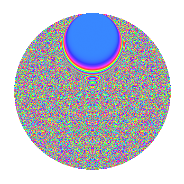# Properties

 Label 2304.2.caLevel $2304$ Weight $2$ Character orbit 2304.ca Rep. character $\chi_{2304}(13,\cdot)$ Character field $\Q(\zeta_{192})$ Dimension $24448$ Sturm bound $768$

# Related objects

## Defining parameters

 Level: $$N$$ $$=$$ $$2304 = 2^{8} \cdot 3^{2}$$ Weight: $$k$$ $$=$$ $$2$$ Character orbit: $$[\chi]$$ $$=$$ 2304.ca (of order $$192$$ and degree $$64$$) Character conductor: $$\operatorname{cond}(\chi)$$ $$=$$ $$2304$$ Character field: $$\Q(\zeta_{192})$$ Sturm bound: $$768$$

## Dimensions

The following table gives the dimensions of various subspaces of $$M_{2}(2304, [\chi])$$.

Total New Old
Modular forms 24704 24704 0
Cusp forms 24448 24448 0
Eisenstein series 256 256 0

## Trace form

 $$24448q - 32q^{2} - 64q^{3} - 32q^{4} - 32q^{5} - 64q^{6} - 32q^{7} - 128q^{8} - 64q^{9} + O(q^{10})$$ $$24448q - 32q^{2} - 64q^{3} - 32q^{4} - 32q^{5} - 64q^{6} - 32q^{7} - 128q^{8} - 64q^{9} - 128q^{10} - 32q^{11} - 64q^{12} - 32q^{13} - 32q^{14} - 64q^{15} - 32q^{16} - 128q^{17} - 64q^{18} - 128q^{19} - 32q^{20} - 64q^{21} - 32q^{22} - 32q^{23} - 64q^{24} - 32q^{25} - 128q^{26} - 64q^{27} - 128q^{28} - 32q^{29} - 64q^{30} - 32q^{31} - 32q^{32} - 64q^{33} - 32q^{34} - 128q^{35} - 64q^{36} - 128q^{37} - 32q^{38} - 64q^{39} - 32q^{40} - 32q^{41} - 64q^{42} - 32q^{43} - 128q^{44} - 64q^{45} - 128q^{46} - 32q^{47} - 64q^{48} - 32q^{49} - 32q^{50} - 64q^{51} - 32q^{52} - 128q^{53} - 64q^{54} - 128q^{55} - 32q^{56} - 64q^{57} - 32q^{58} - 32q^{59} - 64q^{60} - 32q^{61} - 128q^{62} - 64q^{63} - 128q^{64} - 32q^{65} - 64q^{66} - 32q^{67} - 32q^{68} - 64q^{69} - 32q^{70} - 128q^{71} - 64q^{72} - 128q^{73} - 32q^{74} - 64q^{75} - 32q^{76} - 32q^{77} - 64q^{78} - 32q^{79} - 128q^{80} - 64q^{81} - 128q^{82} - 32q^{83} - 64q^{84} - 32q^{85} - 32q^{86} - 64q^{87} - 32q^{88} - 128q^{89} - 64q^{90} - 128q^{91} - 32q^{92} - 64q^{93} - 32q^{94} - 32q^{95} - 64q^{96} - 32q^{97} - 128q^{98} - 64q^{99} + O(q^{100})$$

## Decomposition of $$S_{2}^{\mathrm{new}}(2304, [\chi])$$ into newform subspaces

The newforms in this space have not yet been added to the LMFDB.